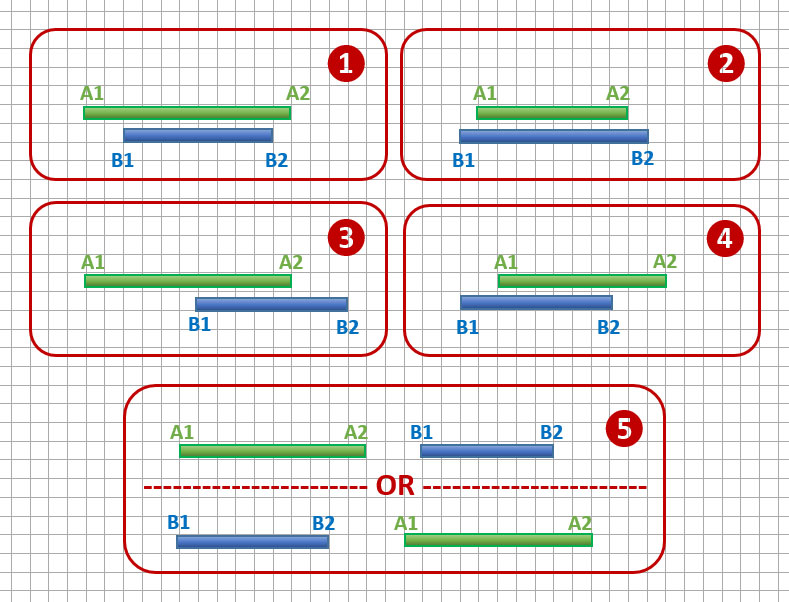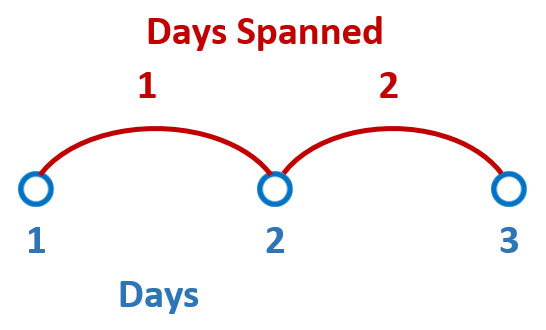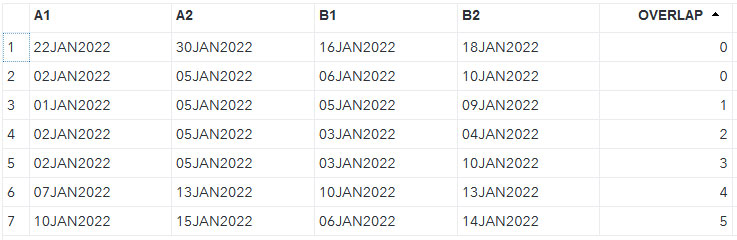In this post, we tackle the problem of calculating the length of overlap of two date/time intervals. For example, we might have two events — each lasting several days — and need to calculate the number of shared days between both events. In other words, we need to calculate the length of the events’ overlap.

Such tasks are typical in clinical trials programming, health (and non-health) insurance claim processing, project management, planning and scheduling, etc.

The length of the overlap can be measured not only in days, but also in any other units of the date/time dimension: hours, minutes, seconds, weeks, months, years and so on. Moreover, the date/time application is just one special case of a broader task of calculating the overlap length of two integer intervals. An integer interval [x .. y] is a set of all consecutive integer numbers beginning with x and ending with y (boundaries included and x ≤ y).

Instead of suggesting a single “best” way of solving this problem, I offer three different strategies. This allows you to compare and weigh their pros and cons and decide for yourself which approach is most suitable for your circumstances. All three solutions presented in this blog post are applicable to the date/time use case, as well as its superset of integer intervals’ overlap length calculation.

## Problem description

Suppose we have two events A [A1 .. A2] and B [B1 .. B2]. Event A lasts from date A1 until date A2 (A1 ≤ A2), and event B lasts from date B1 until date B2 (B1 ≤ B2).  Both starting and ending dates are included in the corresponding event intervals.

We need to calculate the overlap between these two events, defined as the number of days that belong to both events A and B.

## Overlapping intervals: sample data

Before solving the problem, let’s create sample data to which we are going to apply our possible solutions.

The following data step creates data set EVENTS representing such a data sample:

```data EVENTS; input A1 A2 B1 B2; format A1 A2 B1 B2 date9.; informat A1 A2 B1 B2 mmddyy10.; lines; 01/02/2022 01/05/2022 01/06/2022 01/10/2022 01/22/2022 01/30/2022 01/16/2022 01/18/2022 01/02/2022 01/05/2022 01/03/2022 01/10/2022 01/02/2022 01/05/2022 01/03/2022 01/04/2022 01/10/2022 01/15/2022 01/06/2022 01/14/2022 01/01/2022 01/05/2022 01/05/2022 01/09/2022 01/07/2022 01/13/2022 01/10/2022 01/13/2022 ;```

Now let’s go over the following three distinct possible solutions, each with its own merit and downside. At the end, you decide which solution you like the most.

## Solution 1: Brute force

In general, brute force algorithms solve problems by exhaustive iteration through all possible choices until a solution is found. It rarely results in a clever, efficient solution. However, it is a straightforward, valid and important algorithm design strategy that is applicable to a wide variety of problems.

For integer intervals overlap calculation, a brute force approach would involve the following steps:

1. Determine the earliest date in the definitions of our two intervals
2. Determine the latest date in the definitions of our two intervals
3. Create an iterative loop with index variable ranging from the earliest to the latest date
4. Within this loop, increment a counter by one if index variable falls within both intervals
5. At the end of this loop, the counter will equal the desired value for the overlap.

Here is the code implementation of the described above algorithm:

```data RESULTS; set EVENTS; OVERLAP = 0; do i=min(A1,B1) to max(A2,B2); if (A1<=i<=A2) and (B1<=i<=B2) then OVERLAP + 1; end; run;```

As you can see, the code implementation of the brute force solution is quite simple. However, it is hardly efficient as it involves extensive looping.

## Solution 2: Exhaustive logic

Exhaustive logic algorithms solve problems by splitting them into several smaller problems (logical units or classes). This way, instead of working on a problem in its entirety, you can work separately on smaller and simpler problems that are easier to comprehend, digest and solve.

Here is an illustration of various arrangements of integer intervals representing separate logical units (numbered 1...5) when calculating the intervals overlaps:The following SAS code demonstrates an implementation of the exhaustive logic algorithm (each IF-THEN statement corresponds to the numbered logical unit in the above figure):

```data RESULTS; set EVENTS; if A1<=B1 and A2>=B2 then OVERLAP = B2 - B1 + 1; else if A1>=B1 and A2<=B2 then OVERLAP = A2 - A1 + 1; else if A1<=B1<=A2 and B2>A2 then OVERLAP = A2 - B1 + 1; else if B1<=A1<=B2 and A2>B2 then OVERLAP = B2 - A1 + 1; else if B1>A2 or A1>B2 then OVERLAP = 0; run;```

Notice that we need to add 1 to each of the two days difference. That is because the number of days spanned is always 1 less than the number of days contained in an interval. Here is an illustration:## Solution 3: Holistic Math

Finally, a holistic math method looks at the problem in its entirety and condenses it to a comprehensive mathematical formula. While it is usually not the most obvious approach, and often requires some brain stretching and internalization, the effort is often rewarded by achieving the best possible solution.

The exhaustive logic solution may help in better understanding the problem and arriving at the generalized mathematical formula.

If you dwell on the exhaustive logic solution for a while, you may come to realization that all the variety of the logical units boils down to the following single simple formula:

Overlap = min(A2, B2) - max(A1, B1) + 1.

In other words, the overlap of two integer intervals is a difference between the minimum value of the two upper boundaries and the maximum value of the two lower boundaries, plus 1.

Positive OVERLAP value represents actual overlap days, while zero or negative value means that intervals do not overlap at all. The higher absolute value of the negative result – the farther apart the intervals are.  To correct this “negativity” effect we can just set all negative values to zero.

The following SAS code implements the described holistic math approach:

```data RESULTS; set EVENTS; OVERLAP = min(A2,B2) - max(A1, B1) + 1; if OVERLAP<0 then OVERLAP = 0; run;```

As you can see, this in the most concise, elegant, and at the same time the most efficient solution with no iterative looping and complicated exhaustive (and exhausting) logic.

## Output

Here is the output data table RESULTS showing our sample intervals (columns A1, A2, B1 and B2) and the resulting column OVERLAP (all three solutions described above should produce identical OVERLAP values):